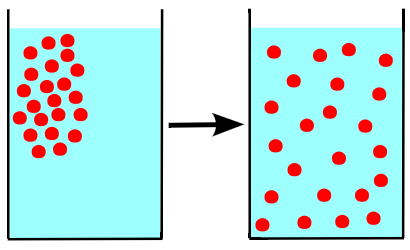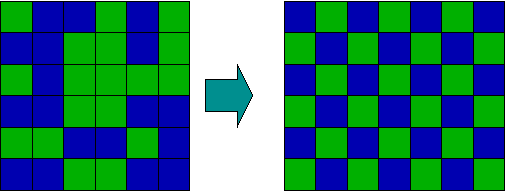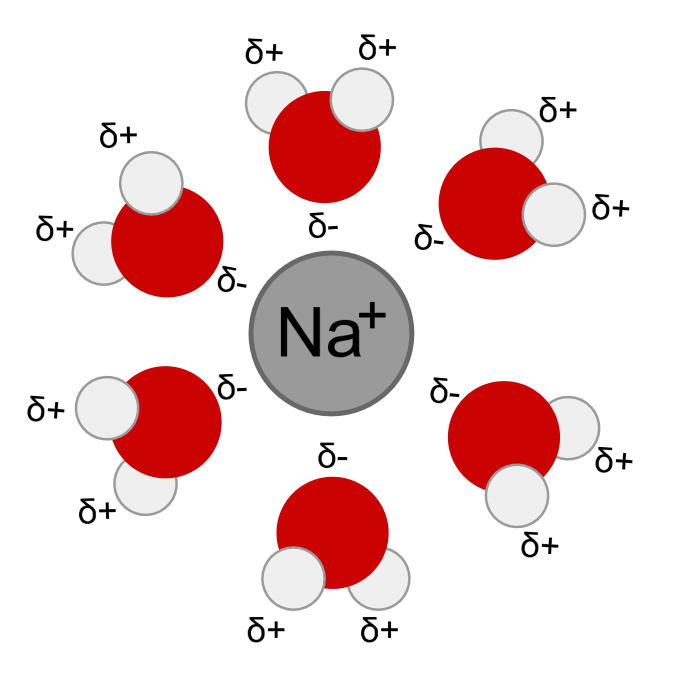## Intermolecular Forces and Solutions

To form a solution, molecules of solute and solvent must be more attracted to each other than themselves.

### Learning Objectives

Recall the two conceptual steps necessary to dissolve a solute and form a solution

### Key Takeaways

#### Key Points

• There are two conceptual steps to form a solution, each corresponding to one of the two opposing forces that dictate solubility.
• The first conceptual step is dissolution, which corresponds to the force of the solvent -solvent and solute -solute intermolecular attractions that needs to be broken down.
• The second conceptual step is solvation, which corresponds to the force of the solute-solvent intermolecular attraction that needs to be formed in order to form a solution.
• Many intermolecular forces can contribute to solvation, including hydrogen bonding, dipole -dipole forces, Van Der Waals forces, and ion -dipole interactions.

#### Key Terms

• intermolecular forces: attractive and repulsive forces between molecules

The strength of the intermolecular forces between solutes and solvents determines the solubility of a given solute in a given solvent. In order to form a solution, the solute must be surrounded, or solvated, by the solvent. Solutes successfully dissolve into solvents when solute-solvent bonds are stronger than either solute-solute bonds or solvent-solvent bonds.

Qualitatively, one can determine the solubility of a solute in a solvent by using the rule “like dissolves like”. In general, solutes whose polarity matches that of the solvent will generally be soluble. For example, table salt (NaCl) dissolves easily into water (H2O) because both molecules are polar.

### Intermolecular Forces and Their Importance in Solution Formation

There are two conceptual steps to form a solution, each corresponding to one of the two opposing forces that dictate solubility. If the solute is a solid or liquid, it must first be dispersed — that is, its molecular units must be pulled apart. This requires energy, and so this step always works against solution formation (always endothermic, or requires that energy be put into the system).Step 1 of dissolution: Molecules going from an ordered state, such as a solid, to a disordered state require an input of energy.The nature of the solute (X) and solvent (Y) determines whether dissolution is energetically favorable or unfavorable. If the solute binds to other solute (X-X bond) more strongly than the solute binds to the solvent (X-Y bond), then the dissolution is not energetically favorable.

### Step 1: Dissolving Solutes

The nature of the solute (X) and solvent (Y) determines whether dissolution is energetically favorable or unfavorable. If the solute binds to other solute (X-X bond) more strongly than the solute binds to the solvent (X-Y bond), then the dissolution is not energetically favorable.

On the other hand, dissolution is favorable when solute-solvent bonds (X-Y) are stronger than X-X or Y-Y bonds. In this case, the potential energy is lower when the solute and solvent can form bonds. If the X-Y attractions are stronger than the X-X or Y-Y attractions, the dissolution reaction is exothermic and releases energy when the solute and solvent are combined.

Since the dissolution of the solvent (X-X) and solute (Y-Y) is always positive, the determining factor for solution formation is the value of X-Y. Remember that the interactions between X and Y, represented above as X-Y, are classified as intermolecular forces, which are not covalent (bonding) interactions.

### Step 2: Forming a Solution

After dissolution occurs, solvation follows. If solvation releases more energy than is consumed during dissolute, then solution formation is favored and the solute is soluble in the solvent. Many intermolecular forces can contribute to solvation, including hydrogen bonding, dipole-dipole forces, and Van Der Waals forces.

### Ion-Dipole Interactions

Another common example of these forces at work is an ion-dipole interaction, which arises when water solvates ions in solution. This interaction arises most prevalently when strong or weak electrolytes are place in water. Consider the dissolution of table salt (sodium chloride) in water:

$\text{NaCl} (\text{s}) \rightarrow \text{Na}^+(\text{aq})+\text{Cl}^-(\text{aq})$

The water molecules form a solvent cage around each Na+ or Cl ion, as implied by the aqueous state symbol (aq) following each of the products. The positive ion, Na+, is surrounded by water molecules that have the negative dipoles of the water, or the oxygen, pointing towards the cation.Solvation of a cation by water.: Water molecules (gray/green is hydrogen, orange is oxygen) surround a sodium cation in a solution. Notice the negative dipole or the oxygen molecules are ‘facing’ the Na+.

In this case, the anion Cl is solvated by the positive dipoles of water, which are represented by hyrogen atoms. In general, when ions are present in water, each cation and anion is surrounded by a ‘cage’ of partial negative or partial positive charge, respectively. These interactions explain why most ionic compounds are considered soluble in water, unless specifically labeled otherwise.

## Solutions and Entropy Changes

An increase in entropy occurs when a solution is formed, providing one of the many driving forces for this process.

### Learning Objectives

Recall that entropy favors dissolution because the potential for randomness is increased

### Key Takeaways

#### Key Points

• Entropy can be thought of as the randomness or spread-outedness of a group of molecules. Increasing randomness is favorable.
• There is an entropy change associated with the formation of a solution, an increase in entropy (randomness) that thermodynamically favors the solution over the two original states.
• If the other energetics of dissolution are favorable, this increase in entropy means that the conditions for solubility will always be met. Even if the energetics are slightly endothermic the entropy effect can still allow the solution to form.

#### Key Terms

• entropy: A thermodynamic property that is the measure of a system’s thermal energy per unit temperature that is unavailable for doing useful work.

### Entropy

As anyone who has shuffled a deck of cards knows, disordered arrangements of objects are statistically more favored, simply because there are more ways in which they can be realized. The more the number of objects increases, the more statistics governs their most likely arrangements. Chemistry deals with a huge number of objects (molecules), and their tendency to become as spread out and disordered as possible can become overwhelming. However, when they become spread out and disordered, the thermal energy they carry with them is also dispersed; the availability of this energy as measured by the temperature is also of importance. Chemists use the term “entropy” to denote this aspect of molecular randomness. Entropy is indeed a fascinating, but somewhat confusing, topic. In fact, it is so important that the topic of entropy deals with two of the three laws of thermodynamics.Order and disorder: This image shows a series of blue and green squares going from a state of disorder (randomness) to a state of order (a clear repeating pattern). In this example, that is to say, entropy decreases and opposes the transition.

### Entropy in Solution Formation

For now, entropy can be thought of as molecular “disorder” or in terms of the energy of molecules and how spread out they are. This term increases with increasing temperature. As a molecule changes state, the general states of matter can be ordered as follows in terms of entropy: gases > liquids > solids.

In a similar manner entropy plays an important role in solution formation. Entropy commonly increases especially for ions as they transition from molecule to ions. This is because we are essentially increasing the number of particles from one compound to two or more depending upon the composition. Consider, the dissolution of sodium sulfate,

Na2SO4(s) –> 2 Na+ (aq) + SO42-(aq)

The entropy is increasing for two reasons here:

1. A solid is formed into aqueous media.
2. One molecule is transformed into three ions.

All these factors increase the entropy of the solute. Also keep in mind that there is a loss of entropy associated with the water moleclues organizing their ‘ solvent cages’ around the ions themselves. This factor can sometimes lead to only a small increase in entropy although a large increase is expected. Thus, in the very common case in which a small quantity of solid or liquid dissolves in a much larger volume of solvent, the solute becomes more spread out in space, and the number of equivalent ways in which the solute can be distributed within this volume is greatly increased. This is the same as saying that the entropy of the solute increases.

Think of entropy in solution formation by picturing the addition of food coloring to pure water. Upon first addition of the food coloring, the dye molecules are concentrated near their contact point. As time proceeds, these molecules of dye are dispersed more uniformly throughout the solution even without mixing. Since the Hsolution for this process is approximately zero (an ideal solution), the only thermodynamic factor driving the mixing is the entropy term.

Diffusion: Water & Food Dye – Diffusion Project: When food dye is added to water, a solution is formed. This formation of solution increases entropy as the molecules become more evenly distributed and ordered through the process of diffusion.

If the energetics of dissolution are favorable, this increase in entropy means that the conditions for solubility will always be met. Even if the energetics are slightly endothermic, the entropy effect can still allow the solution to form, although they may perhaps limit the maximum concentration that can be achieved.

## Solutions and Heats of Hydration

When ions dissolve in water, the stabilizing interactions that result release energy called the “heat of hydration.”

### Learning Objectives

Predict whether a given ionic solid will dissolve in water given the lattice energy and heat of hydration

### Key Takeaways

#### Key Points

• In order to dissolve an ionic solid, water molecules must break up the interactions between all of the ions in the solid. To do this, they orient themselves such that they effectively reduce the localized charge on the ions. This is called hydration.
• Hydration of ions is a thermodynamically favorable process, and as such can release heat. This is why it is called the ” heat of hydration.”
• The heat of hydration (Hhydration) offsets the lattice energy (Hlattice energy) of an ionic solid to allow for solution formation to occur typically when Hhydration > Hlattice energy.

#### Key Terms

• thermodynamics: The science of the conversions between heat and other forms of energy.
• ion: An atom or group of atoms bearing an electrical charge, such as the sodium and chlorine atoms in a salt solution.
• heat of hydration: The heat that is released by hydration of one mole of ions at a constant pressure. The more the ion is hydrated, the more heat is released.

### The Energetics of Solution Formation

Solubility depends on dissolution of the solute into the solvent and, like all chemical reactions, is governed by the laws of thermodynamics. This particular process is a change of state from the solute’s starting state, either solid, liquid or gas, to a dissolved state (termed aqueous when the solvent is water), which is a distinct physical state and thus is considered a chemical reaction. In order for any chemical reaction to proceed, it must be thermodynamically favorable. Many factors influence how thermodynamically favorable a given reaction is, including the heat of hydration, or hydration energy released when water solvates, or surrounds, an ion, and the amount of energy required to overcome the attractive forces between solute molecules, known as lattice energy.

### Solvent-Solute Interactions

Since the coulombic forces that bind ions and highly polar molecules into solids are quite strong, we might expect these solids to be insoluble in most solvents. The attractive interactions between ionic molecules are called the lattice energy, and they must be overcome for a solution to form. Ionic solids are insoluble in the majority of non-aqueous solvents, but they tend to have high solubility specifically in water.

The key factor that determines solubility is the interaction of the ions with the solvent. The electrically-charged ions undergo ion- dipole interactions with water to overcome strong coulombic attraction, and this produces an aqueous solution. The water molecule is polar; it has a partial positive charge on the hydrogens while oxygen bears a partial negative charge. This dipole arises from the disparity in electronegativity present in the O-H bonds within the water molecule. Furthermore, the two lone pairs on the oxygen in water also contribute to the stabilization of any positively charged ions in solution.Hydrated Na+H2O cation: Water molecules surround and stabilize a cation via interaction with the partial negative charge on the oxygen ends.

As a consequence, ions in aqueous solutions are always hydrated; that is, they are quite tightly bound to water molecules through ion-dipole interactions. The number of water molecules contained in the primary hydration shell, which completely encompasses the ion, varies with the radius and charge of the ion.

### Lattice Energy

The dissolution of an ionic solid MX in water can be thought of as a sequence of two processes:

$1)<\text{br}>\text{MX} (\text{s}) \to \text{M}^+ (\text{g}) + \text{X}^-(\text{g})$ [lattice energy]

$2.) \text{M}^+ (\text{g}) + \text{X}^-(\text{g}) \to \text{M}^+ (\text{aq}) + \text{X}^-(\text{aq})$ [heat of hydration]

The first reaction (ionization) is always endothermic; it takes a lot of work to break up an ionic crystal lattice into its component ions. Lattice energy is defined as the energy that is released when one mole of ionic solid is formed from gaseous ions, and it increases with increasing atomic charge and decreasing atomic size (radii). The greater the value of a compound ‘s lattice energy, the greater the force required to overcome coulombic attraction. In fact, some compounds are strictly insoluble due to their high lattice energies that cannot be overcome to form a solution.

### Heat of Hydration (Hhydration) vs Lattice Energy

The hydration step in the second reaction is always exothermic (Hhydration < 0) as H2O molecules are attracted into the electrostatic field of the ion. The heat ( enthalpy ) of solution (Hsolution) is the sum of the lattice and hydration energies ( Hsolution = Hhydration + Hlattice energy). From this relationship, we can clearly see that the processes of overcoming the lattice energy and hydrating the ions are in competition with one another.

The value of Hsolution is dependent upon the magnitudes of Hhydration and Hlattice energy of the solute. Favorable conditions for solution formation typically involve a negative value of Hsolution; this arises because the hydration process exceeds the lattice energy in the solute. As often happens for a quantity that is the sum of two large terms having opposite signs, the overall dissolution process can be either endothermic or exothermic. Hsolution is just one of the factors determining solution formation, but it is typically the major consideration in solution formation because of the role that enthalpy plays in most thermodynamic considerations.

The average time an ion spends in a hydration shell is about two to four nanoseconds, which is about two orders of magnitude longer than the lifetime of an individual H2O–H2O hydrogen bond. The relative strengths of these two intermolecular forces is apparent: ion-dipole interactions are stronger than hydrogen bond interactions.

In case you were wondering where we got the term “heat of hydration,” it has to do with the fact that some solutions are highly exothermic when formed. A hot solution results when the heat of hydration is much greater than the lattice energy of the solute.Enthalpy diagram for the dissolution process: The enthalpy diagram showing exothermic solution formation. Notice that Hsolution is lower in energy than the starting solute/solvent enthalpies. An endothermic process, on the other hand, would show Hsolution as positive, and it would be higher in energy than the starting solute/solvent enthalpies.